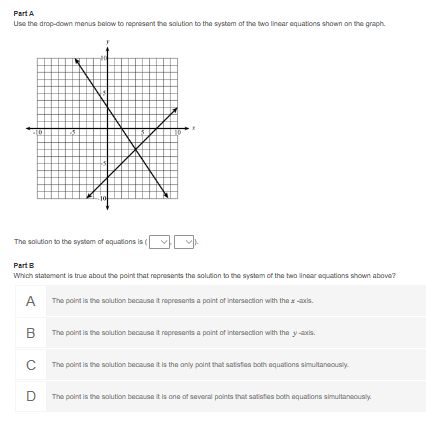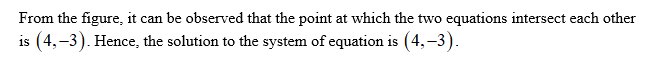# Part AUse the drop-down manus below to represent the solution to the system at the two linear aquations shown an the graph.The solution to the system of equations is (Part BWhich statement is true about the point that rapresents the solution to the system of the two linaar equations shown above?A The point is the solution becsuse it represents a point of intersection with the z -axis.The point is the solution because it represents a point of intersection with the y-axis.C The point is the solution because it is the anly point that satisfas both aquations simultaneously.D The point is the solution because it is ona of saveral points that satistias both aquations simultanaously.

Question
1 viewshelp_outlineImage TranscriptionclosePart A Use the drop-down manus below to represent the solution to the system at the two linear aquations shown an the graph. The solution to the system of equations is ( Part B Which statement is true about the point that rapresents the solution to the system of the two linaar equations shown above? A The point is the solution becsuse it represents a point of intersection with the z -axis. The point is the solution because it represents a point of intersection with the y-axis. C The point is the solution because it is the anly point that satisfas both aquations simultaneously. D The point is the solution because it is ona of saveral points that satistias both aquations simultanaously. fullscreen
check_circle

star
star
star
star
star
1 Rating
Step 1

Given the graph of  the system of two linear equations.

Step 2...

### Want to see the full answer?

See Solution

#### Want to see this answer and more?

Solutions are written by subject experts who are available 24/7. Questions are typically answered within 1 hour.*

See Solution
*Response times may vary by subject and question.
Tagged in